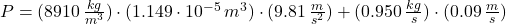## You pull a solid nickel ball with a density of 8.91 g/cm3 and a radius of 1.40 cm upward through a fluid at a constant speed of 9.00 cm/s. T

Question

You pull a solid nickel ball with a density of 8.91 g/cm3 and a radius of 1.40 cm upward through a fluid at a constant speed of 9.00 cm/s. The fluid exerts a drag force that is directly proportional to speed, and the proportionality constant is 0.950 kg/s. What is the magnitude of the force (in N) you exert on the ball? (You may ignore the buoyant force.)

in progress 0
2 weeks 2021-08-28T17:50:41+00:00 1 Answers 0 views 0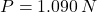Explanation:

The constant speed means that ball is not experimenting acceleration. This elements is modelled by using the following equation of equilibrium: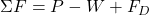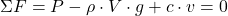Now, the exerted force is:The volume of a sphere is: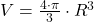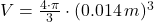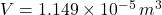Lastly, the force is calculated: